# How to Accurately Calculate Ages in Power BI

### Colin Maitland, 07 October 2019

In this article, I demonstrate how to calculate Ages in whole-years and fractional-years in Power BI using several different approaches. I also identify the approach that calculates the most accurate Age.

The following image shows a list of people in Power BI. These records were loaded into the Power BI data model from the Dynamics 365 Contacts entity. The First Name, Last Name and Date of Birth columns were loaded from Dynamics 365. The Age columns are additional columns that have been added to the Power BI data model. The ages are calculated against the current date; e.g. 19/09/2019, whenever the data model is refreshed. The Total shows the average age for each column.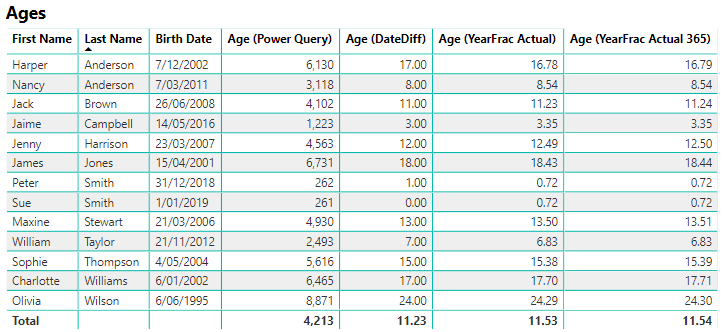The Age (Power Query) column is a custom column added using Power Query. This column shows each person’s age in days based on the current date. This column was added using the Age option under Date on the Add Column tab of the Power Query ribbon bar. The Age is in days.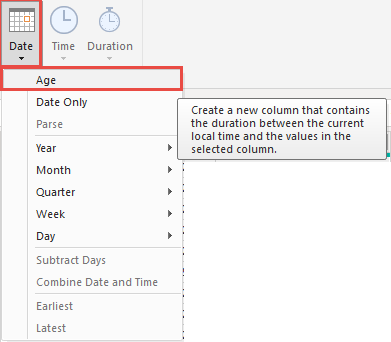The following formula is automatically generated and resulting in the addition of a custom column: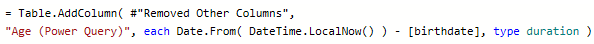The Age (DateDiff) column is a calculated column added using the following DAX, Data Analysis Expression, expressions: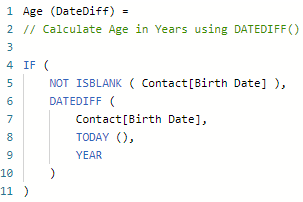This column shows each person’s age in years based on the current date calculated using the DATEDIFF function with its third parameter set to YEAR.

Notice that Peter Smith and Sue Smith, who are twins born a few minutes apart on either side of midnight on 31/12/2018 and 1/01/2019, have calculated ages of 1 and 0 years old respectively.

Peter’s calculated age is not accurate! It should not be 1 until he reaches his first birthday. Sue’s calculated age is more accurate than Peter’s.

The DATEDIFF function, when used in this way counts the number of transitions from one year to another between two dates. A transition from 31/12/2018 to 1/01/2019 returns 1, even though only one day has elapsed.

For each person, including the others in the list, this calculation also does not account for fractional years.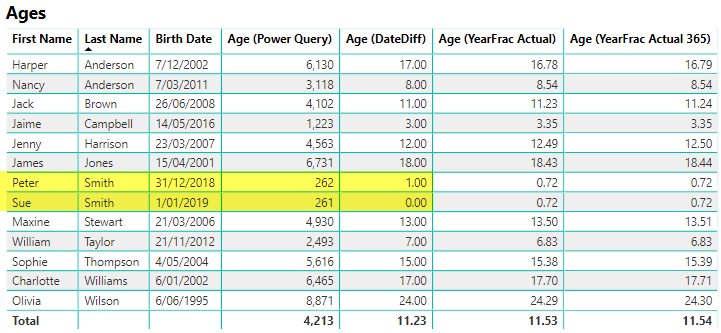The Age (YearFrac Actual) and Age (YearFrac Actual 365) columns both calculated columns added using the following DAX expressions: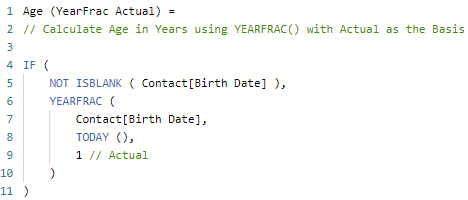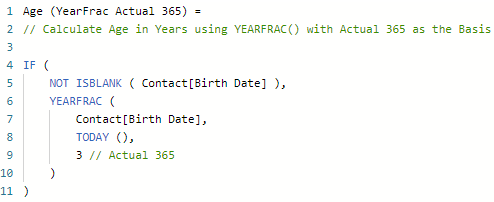These two variations of using the YEARFRAC function calculate very similar results and account for fractional years.

When using Actual as the day count basis, the calculation is based on the actual number of days per year; i.e. 366 days for leap years and 365 days for other years. When using Actual 365 as the day count basis, the calculation is based on a 365-day year.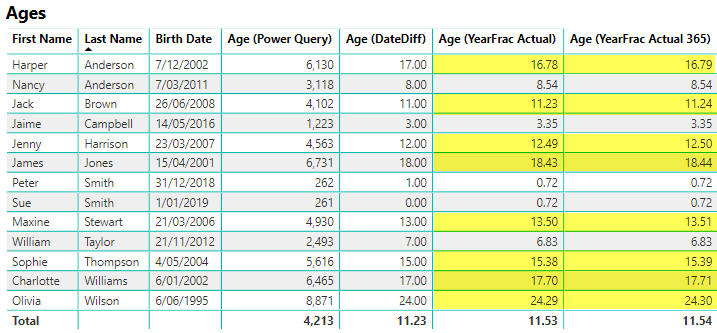If you want to calculate the age in whole-years using a DAX calculated column, the following formula achieves this by using INT to truncate the fractional part.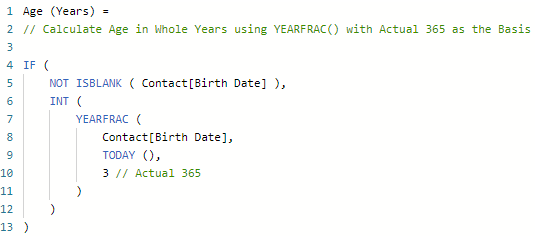The calculated age for each person in whole-years is now correct. The following image highlights those that were previously incorrect.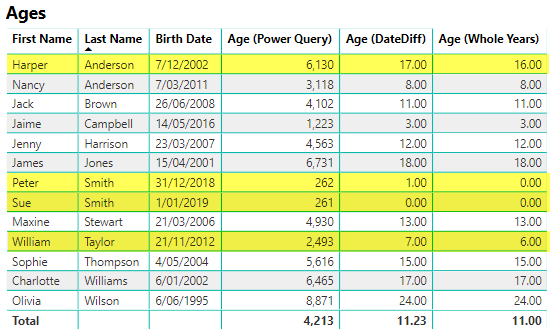Implementing the equivalent logic in Power Query using the following steps does not provide the same results for calculating Ages in whole-Years.

1. Add a custom column named Age using Age under Date on the Transform tab.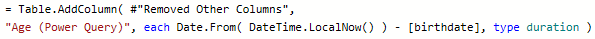1. Transform Age from Days to Years using Total Years under Duration on the Transform tab.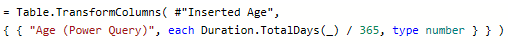1. Transform Age from fractional-Years to whole-Years using Data Type on the Transform tab; i.e. Change the Data Type from Decimal Number to Whole Number.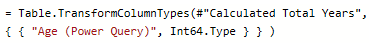In fact, many of the calculated Ages in whole-Years are not correct. The following image highlights those that are not correct.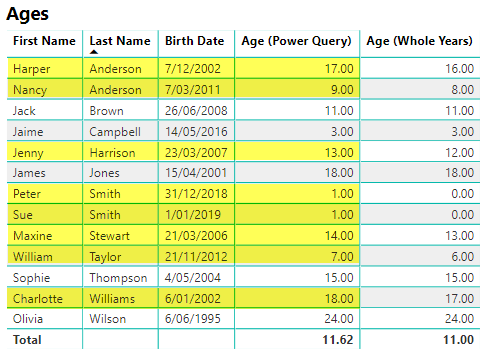Changing the Total Years calculation in Power BI to use 365.25 instead of 360 as the days count basis results only in the calculated Age for Jenny Harrison being corrected; i.e. to from 13 to 12.

In conclusion, the most accurate Age calculation is achieved using the DAX DATEFRAC function.

Finally, be aware of the fact that the DateTime.LocalNow() and Today() functions used in these formulas are based on the system date/time of the workstation or server on which the Power BI data model is refreshed.